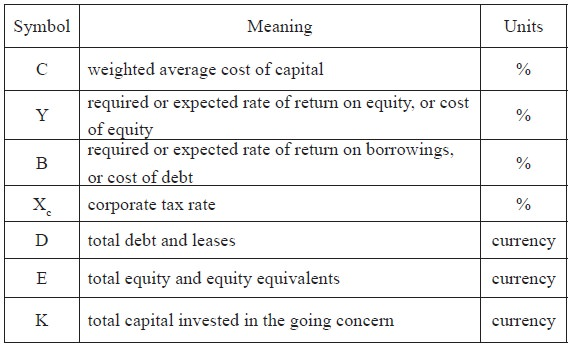Home | ARTS | Financial Management | Weighted average cost of capital - CAPITAL STRUCTURE THEORIES

# Weighted average cost of capital - CAPITAL STRUCTURE THEORIES

Posted On :  20.06.2018 03:43 am

The weighted average cost of capital (WACC) is used in finance to measure a firm’s cost of capital.

Weighted average cost of capital

The weighted average cost of capital (WACC) is used in finance to measure a firm’s cost of capital. It had been used by many firms in the past as a discount rate for financed projects, since using the cost of the financing seems like a logical price tag to put on it.

Companies raise money from two main sources: equity and debt. Thus the capital structure of a firm comprises three main components: preferred equity, common equity and debt (typically bonds and notes). The WACC takes into account the relative weights of each component of the capital structure and presents the expected cost of new capital for a firm

The formula

The weighted average cost of capital is defined by

C = (E/K) y + (D/K) b (1 – Xc)

Where,

K = D + E

The following table defines each symbolThis equation describes only the situation with homogeneous equity and debt. If part of the capital consists, for example, of preferred stock (with different cost of equity y), then the formula would include an additional term for each additional source of capital

## How it works

Since we are measuring expected cost of new capital, we should use the market values of the components, rather than their book values (which can be significantly different). In addition, other, more “exotic” sources of financing, such as convertible/callable bonds, convertible preferred stock, etc., would normally be included in the formula if they exist in any significant amounts - since the cost of those financing methods is usually different from the plain vanilla bonds and equity due to their extra features.

## Sources of Information

How do we find out the values of the components in the formula for WACC? First let us note that the “weight” of a source of financing is simply the market value of that piece divided by the sum of the values of all the pieces. For example, the weight of common equity in the above formula would be determined as follows

Market value of common equity / (Market value of common equity + Market value of debt + Market value of preferred equity)

So, let us proceed in finding the market values of each source of financing (namely the debt, preferred stock, and common stock).

The market value for equity for a publicly traded company is simply the price per share multiplied by the number of shares outstanding, and tends to be the easiest component to find

The market value of the debt is easily found if the company has publicly traded bonds. Frequently, companies also have a significant amount of bank loans, whose market value is not easily found. However, since the market value of debt tends to be pretty close to the book value (for companies that have not experienced significant changes in credit rating, at least), the book value of debt is usually used in the WACC formula

The market value of preferred stock is again usually easily found on the market, and determined by multiplying the cost per share by number of shares outstanding

Now, let us take care of the costs

Preferred equity is equivalent to perpetuity, where the holder is entitled to fixed payments forever. Thus the cost is determined by dividing the periodic payment by the price of the preferred stock, in percentage terms

The cost of common equity is usually determined using the capital asset pricing model

The cost of debt is the yield to maturity on the publicly traded bonds of the company. Failing availability of that, the rates of interest charged by the banks on recent loans to the company would also serve as a good cost of debt. Since a corporation normally can write off taxes on the interest it pays on the debt, however, the cost of debt is further reduced by the tax rate that the corporation is subject to. Thus, the cost of debt for a company becomes (YTM on bonds or interest on loans) × (1 − tax rate). In fact, the tax deduction is usually kept in the formula for WACC, rather than being rolled up into cost of debt, as such

WACC = weight of preferred equity × cost of preferred equity weight of common equity × cost of common equity + weight of debt × cost of debt × (1 − tax rate)
Tags : Financial Management - CAPITAL STRUCTURE THEORIES
Last 30 days 75 views